# To graph: For the function that satisfies the given conditions.### Single Variable Calculus: Concepts...

4th Edition
James Stewart
Publisher: Cengage Learning
ISBN: 9781337687805### Single Variable Calculus: Concepts...

4th Edition
James Stewart
Publisher: Cengage Learning
ISBN: 9781337687805

#### Solutions

Chapter 2, Problem 45RE
To determine

## To graph: For the function that satisfies the given conditions.

Expert Solution

### Explanation of Solution

Given information:

The given conditions f(0)=f'(2)=f'(1)=f'(9) .

Graph:

In the given conditions, the domain is divided into seven intervals: (,2),(2,0),(0,1)(1,6),(6,9),(9,12),(12,) .

In the interval (,2) , the function is decreasing since f'(x)<0 and has positive curvature since f''(x)>0 .

Sketch a line for this part of the function.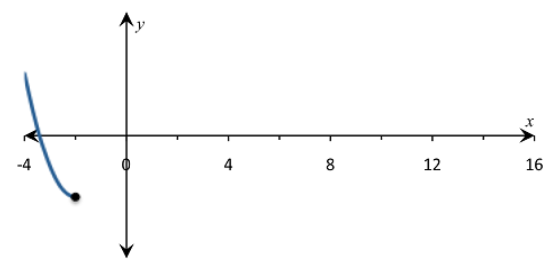Since f(0)=0 , plot the point (0,0) . Then the graph a line for the interval (2,0) .

Since f'(x)>0 , f increasing in this interval. Since f''(x)>0 , it has positive curvature.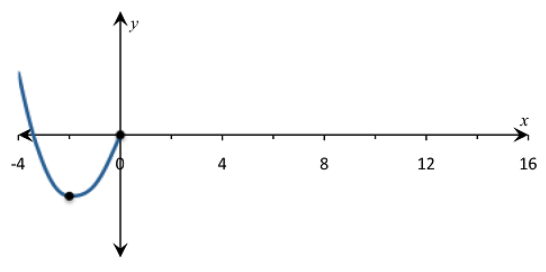In the interval (0,1) , f is increasing since f'(x)>0 and has negative curvature since f''(x)<0 . Sketch a line for this part of function.In the interval (1,6) , the function is decreasing and has negative curvature. In addition, x=6 is an asymptote because limx6f(x)= .In the interval (6,9) , the function is increasing and has negative curvature. It starts from and goes up.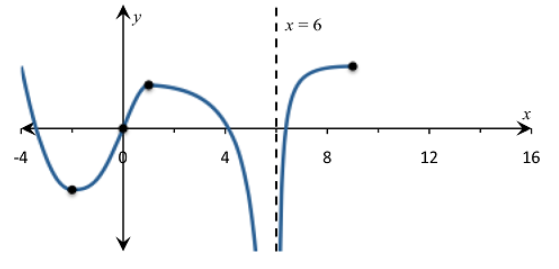In the interval (9,12) , the function is decreasing because f'(x)<0 .

It has negative curvature because f''(x)<0 .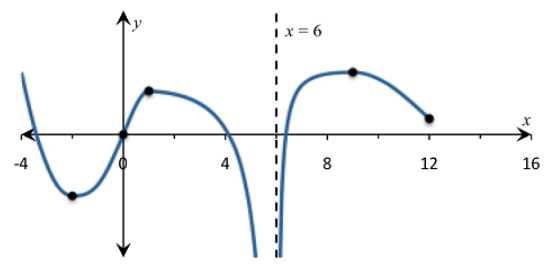Finally, In the last interval (12,) , the function is decreasing and has positive curvature. In addition, the function approaches y=0 from above because limx6f(x)=0 .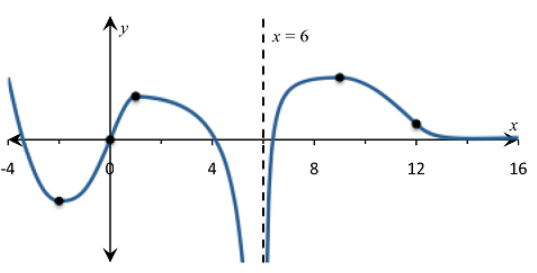Figure (1)

Interpretation: Graph for the function f is shown in figure (1).

### Have a homework question?

Subscribe to bartleby learn! Ask subject matter experts 30 homework questions each month. Plus, you’ll have access to millions of step-by-step textbook answers!